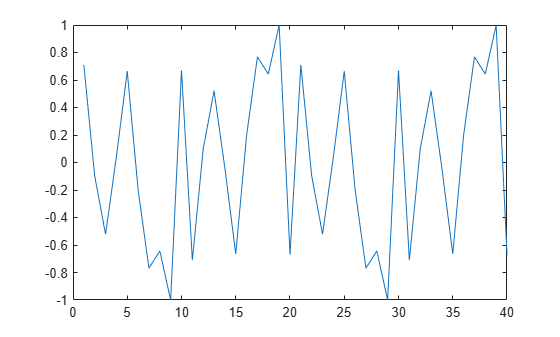# resample

Resample time-domain data that is stored in an `iddata` object by decimation or interpolation (requires Signal Processing Toolbox software)

## Syntax

``datar = resample(data,P,Q)``
``datar = resample(data,P,Q,order)``

## Description

example

````datar = resample(data,P,Q)` resamples the data in `data` such that the `data` is interpolated by a factor `P` and then decimated by a factor `Q`. For example, the command `resample(z,1,Q)` results in decimation by a factor `Q`.```
````datar = resample(data,P,Q,order)` filters the data by applying a filter of specified `order` before interpolation and decimation.```

## Examples

collapse all

Increase the sampling rate of data and compare the resampled and the original data signals.

Use `idinput` to generate an input signal `u` that contains 2 periods, each containing 20 samples, of a sum-of-sinusoids signal with 5 sinusoids.

```u = idinput([20 1 2],'sine',[],[],[5 10 1]); plot(u)```Repackage `u` into an `iddata` object `ui`. Set the start time to `0`. A sum-of-sinusoids signal is band limited, so set `'intersample'` to `'bl'`.

`ui = iddata([],u,1,'tstart',0,'intersample','bl');`

Resample the data using factors of 25 and 3 for `P` and `Q`, respectively. These values produce an increase in sample rate of about 8.3.

```ur = resample(ui,25,3); plot(ui,ur) legend('ui','ur')```The resampled signal is smoother than the original signal.

## Input Arguments

collapse all

Original data, specified as an `iddata` that contains either input/output data or time series data. `data` must contain uniform time samples.

Resampling factor terms, specified as integers that represent the interpolation and decimation factors. After resampling, the new sample time is `Q/P` times the original sample time.

Setting `(Q/P)>1` results in decimation and setting `(Q/P)<1` results in interpolation.

Order of the FIR filter that `resample` applies before interpolation and decimation, specified as a positive integer.

## Output Arguments

collapse all

Resampled data, returned as an `iddata` that contains the same type of data as `data`. The length of the data in `datar` is equal to `P/Q` times the length of the data in `data`.

## Algorithms

`resample` calls the `resample` (Signal Processing Toolbox) function in Signal Processing Toolbox™. The algorithm takes into account the intersample characteristics of the input signal that are described by the `data.InterSample` property.

If you do not have a license for Signal Processing Toolbox, perform resampling using `idresamp`.

## Version History

Introduced before R2006a